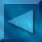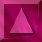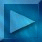Previous : Log-Likelihood derivatives  Next : The modified Geman & Yang... Notations

 Sampling the densities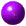Prior model :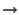First order neighbourhoodModified Geman & Yang sampler, or Gibbs or Metropolis samplers

Examples of a priori samples :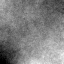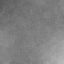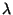=0.3,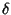=10=1,=15=1,=5Posterior distribution :Data dependence : observed image YHigh order neighbourhood (depends on the size of H)Only the modified Geman & Yang sampler is possible
(Gibbs or Metropolis are too time consuming because of the high order neighbourhood)

Examples of a posteriori samples :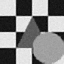=0.5,=10=0.5,=1=10,=10

 Observation image Y :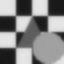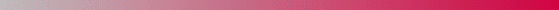André Jalobeanu - 24 Aug 1998